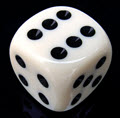# 随机变量： 平均、方差 和 标准差

### 例子：抛硬币：结果可以是正面或反面。• 有个实验（比方抛硬币）
• 我们给每个事件分派数值
• 数值的集合是个随机变量

## 平均、方差和标准差### 例子：抛一个不公平的骰子

 1 2 3 4 5 6 0.1 0.1 0.1 0.1 0.1 0.5

### 平均或期望值：μ

μ = Σxp

• 把每个数值乘以其概率
• 把结果加起来### 例子（续）：

 x 1 2 3 4 5 6 p 0.1 0.1 0.1 0.1 0.1 0.5 xp 0.1 0.2 0.3 0.4 0.5 3

μ = Σxp = 0.1+0.2+0.3+0.4+0.5+3 = 4.5

### 方差：Var(X)

Var(X) = Σx2p − μ2

• 把每个数值的平方乘以其概率
• 把结果加起来：Σx2p
• 减去期望值的平方 μ2### 例子（续）：

 x 1 2 3 4 5 6 p 0.1 0.1 0.1 0.1 0.1 0.5 x2p 0.1 0.4 0.9 1.6 2.5 18

Σx2p = 0.1+0.4+0.9+1.6+2.5+18 = 23.5

Var(X) = Σx2p − μ2 = 23.5 - 4.52 = 3.25

### 标准差：σ

σ = √Var(X)### 例子（续）：

 x 1 2 3 4 5 6 p 0.1 0.1 0.1 0.1 0.1 0.5 x2p 0.1 0.4 0.9 1.6 2.5 18

σ = √Var(X) = √3.25 = 1.803...

（注意这次的列表是垂直排列的。）### 你打算开一家麦德劳炸鸡店。这是市场调查数据：

20% ￥50,000 亏蚀
30% ￥0
40% ￥50,000 利润
10% ￥150,000 利润

xpx2p 的总和：

p

x

xp

x2p
0.2 -50 -10 500
0.3 0 0 0
0.4 50 20 1000
0.1 150 15 2250
Σp = 1   Σxp = 25 Σx2p = 3750

μ = Σxp = 25

Var(X) = Σx2p − μ2 = 3750 − 252 = 3750 − 625 = 3125

σ = √3125 = 56（到最近的整数）

• μ = ￥25,000
• σ = ￥56,000

### 例子（续）：

p

x

xp

x2p
0.1 -50 -5 250
0.1 0 0 0
0.7 50 35 1750
0.1 150 15 2250
Σp = 1 Sums: Σxp = 45 Σx2p = 4250

μ = Σxp = 45

Var(X) = Σx2p − μ2 = 4250 − 452 = 4250 − 2025 = 2225

σ = √2225 = 47（到最近的整数）

• μ = ￥45,000
• σ = ￥47,000

## 连续

• 离散数据只能取某些数值（例如 1、2、3、4、5）
• 连续数据可以取一个范围（值域）里的任何数值（例如人的身高）

## 总结

• A 随机变量是随机实验结果的可能数值。
• 平均（期望值）是：μ = Σxp
• 方差是：Var(X) = Σx2p − μ2
• 标准差是：σ = √Var(X)